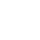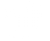# sed Commands Cheat Sheet

• Updated January 10, 2019

`sed` is an extremely powerful stream editor. The web is full of useful sed one liners.

This post will be an ever growing list of `sed` one liners I have found useful.

## Find and Replace

Find and remove all instances of TEXT:

``````sed 's/TEXT//g'
``````

Find and remove all spaces:

``````sed -e 's/ //g'
``````

## Delete all blank lines from a file

``````sed '/^\$/d' <FILE>
``````

## Delete leading whitespace from front of each line

``````sed 's/^[ \t]*//' <FILE>
``````

## Delete trailing whitespace from end of each line

``````sed 's/[ \t]*\$//' <FILE>
``````

## Delete leading and trailing whitespace from each line

``````sed 's/^[ \t]*//;s/[ \t]*\$//' <FILE>
``````

## Insert a line of text before a line

``````sed -i '/PATTERN/i REPLACEWITH' <FILE>
``````

## Change configuration file values

Change BRIDGE_HOTPLUG=yes to BRIDGE_HOTPLUG=no no matter what it is already set to:

``````sed '/BRIDGE_HOTPLUG=/ s/=.*/=no/' /etc/default/bridge-utils
``````

``````sed '/PermitRootLogin / s/ .*/ yes/' /etc/ssh/sshd_config
``````

## Wrap Value in Double Quotes

Change date=2015 to date="2015":

``````echo 'date=2015' | sed 's/\(=[[:blank:]]*\)\(.*\)/\1"\2"/'
``````

## Remove word wrap

``````sed -e :a -e '\$!N;s/\n //;ta' -e 'P;D' <FILE>
``````
``````sed -n 's/.*href="\([^"]*\).*/\1/p' <FILE.html>
``````

## Lower case all href="*" tags

``````sed -e 's/\(href="\([^"]*\)"\)/\L\1/' <FILE>
``````

## Lower case all img src="*" tags

``````sed -e 's/\(img src="\([^"]*\)"\)/\L\1/' <FILE>
``````

## Lower case all href="" tags but exclude href="http" tags

``````sed -e '/\(href="http"*\)/!s/\(href="\([^"]*\)"\)/\L\1/' <FILE>
``````

## Lower case all img src="" tags but exclude img src="http" tags

``````sed -e '/\(img src="http"*\)/!s/\(img src="\([^"]*\)"\)/\L\1/' <FILE>
``````

## Remove '%20' only from lines matching href="*"

``````sed -e '/\(href="\([^"]*\)"\)/s/%20//g' <FILE>
``````top tags (all tags)

computers (162)

documentation (154)

openstack (21)

linux (18)

cheat-sheet (16)

shell (16)

os-x (15)

vagrant (11)

apple (10)

ubuntu (10)